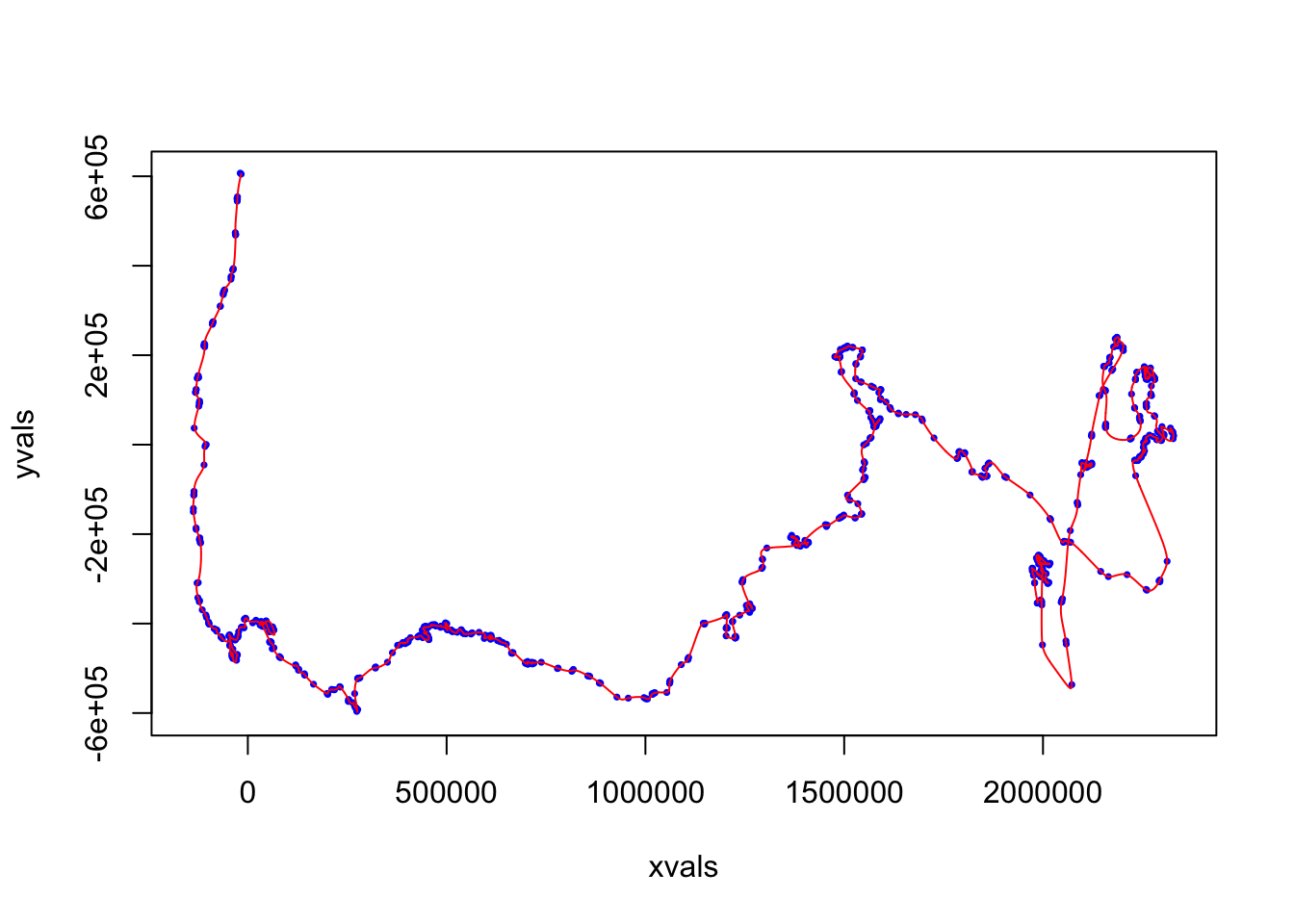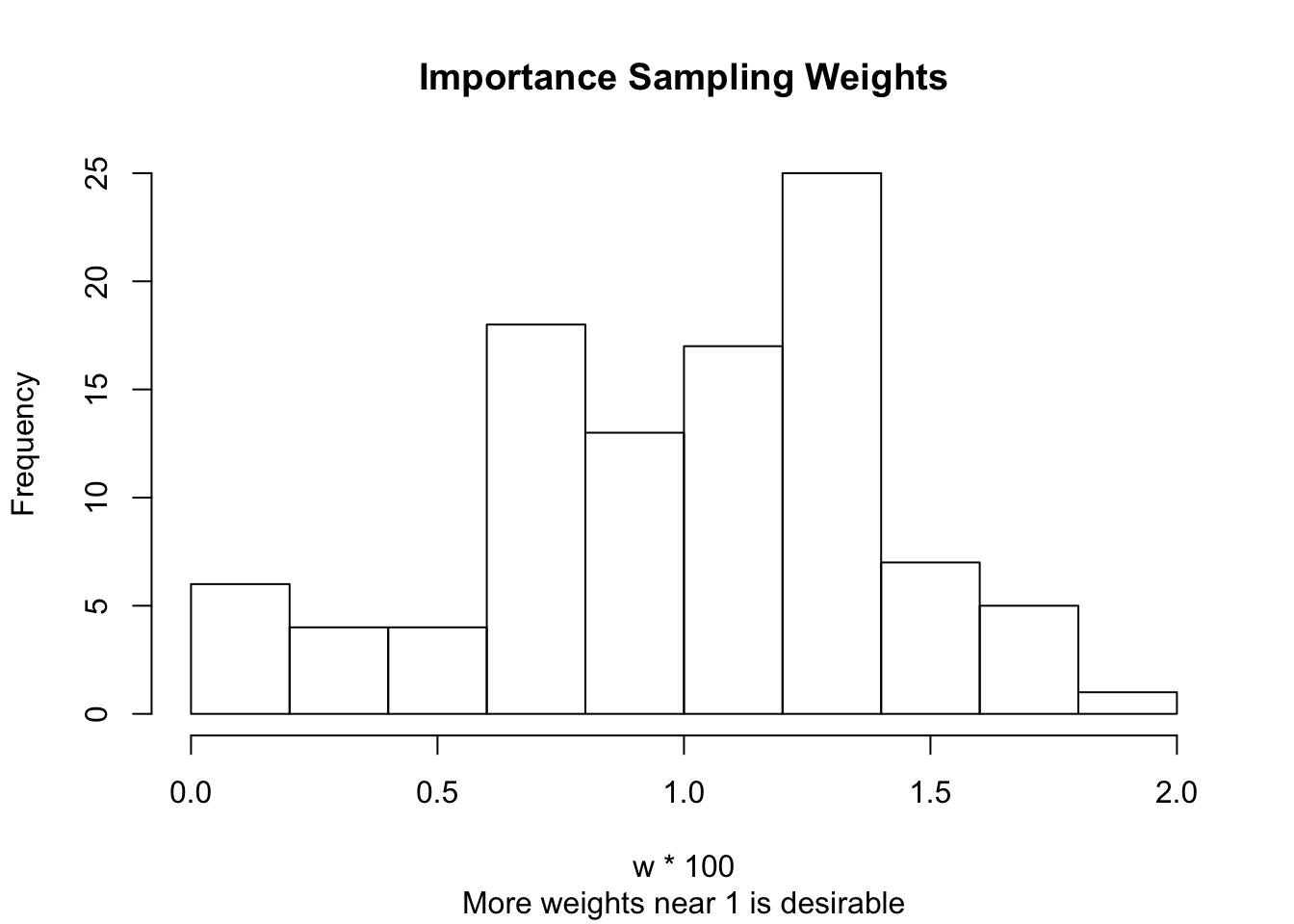# Introduction

Electronic data collection via telemetry instruments is common for many species. Location estimates for the animals are not evenly-spaced in time due to the movement of satellites and the behavior of the animals themselves. In order to view a more continuous representation of the animals’ movement, one must re-create the movement path. Several methods are available to interpolate the movement path, in the Correlated RAndom Walk Library (crawl) we use continuous-time correlated random walk models with time-indexed covariates. The model is fit using a Kalman filter on a state-space version of the continuous-time stochastic movement process.

Telemetry data transmitted via Argos typically have error associated with each location estimate. crawl is now able to incorporate the new error ellipses as well as the older location class designations.

# Preliminary Procedures

To use the package, you must first load the library:

library(crawl)

## Data Preparation

Telemetry data are often downloaded in the proprietory format of the tag manufacturer. Consequently, to run crawl, the data might need to be adjusted. Dataframes must be in a specific format in order for the functions to read the data properly.

Run the following checks on your data prior to building a crawl model:

• Time

The date/time stamp must not contain any missing values, it should be ordered, and the class should be either numeric or POSIXct. To convert a timestamp of the form (“2011-03-27 01:30:00”) to POSIX the following code may be helpful:

as.POSIXct(strptime("2011-03-27", "%Y-%m-%d %H:%M:%S"))

Users may also find the functions within the lubridate package also useful. Additionally, the date-time values should all be unique for a given animal. Duplicate date-time values are a known aspect of Argos data and duplicates should either be filtered from the data set or adjusted. Users can consult the make.time.unique() function within the xts package or the adjust.duplicateTimes() function wtihin the trip package in order to adjust any duplicate date-time values by 1 second.

• Latitude and Longitude (X and Y values)

The lat/long coordinates of your locaiton estimates should be numeric or defined as a spatial points data frame. If they are already spatial points, then check the projection to verify it’s accuracy. If they are numeric, then crawl will assume the data are projected.

• Covariates

If you are using covariates in your model, check to see that there are no missing values. You can have missing locations, but not missing covariates. Covariates that will be included in the model itself must be time-indexed.

You can also use the included Shiny App to check your data for any issues. The app will run basic checks on your Time and lat/long columns to make sure they comply with crawl specifications.

## Northern Fur Seal Demo

This is an example using crawl to model the movements of one animal using Argos location classes for location error estimates, and incorporating drift into the movement process. These data represent Northern fur seal pup relocation data that were used in Johnson et al. (2008). The data are for one seal, including 795 observations with 4 variables. Northern fur seal pups travel long distances and may exhibit both directed travel and movement within large-scale ocean currents. Therefore, a varying drift model is included in this example for the mean velocity.

### Load and clean the data

data("northernFurSeal")
head(northernFurSeal)
##         Time Argos_loc_class latitude longitude
## 1  0.8666667               3   57.109   189.708
## 2  0.9833333               1   57.126   189.664
## 3 11.2833333               2   56.679   189.548
## 4 12.0333333               1   56.645   189.526
## 5 12.4666667               1   56.632   189.546
## 6 13.8833333               1   56.597   189.540

Define the location classes as factors

northernFurSeal$Argos_loc_class <- factor(northernFurSeal$Argos_loc_class,
levels=c("3", "2", "1","0","A"))

### Make sure your data is projected

First, tell R which columns represent the coordinates for the data

library(sp)
library(rgdal)
coordinates(northernFurSeal) = ~longitude+latitude

Get the projection information from the data

proj4string(northernFurSeal) <- CRS("+proj=longlat")

Run a spatial transform for map projection. In this example we define a custom projection for the data based on the location of the animals.

northernFurSeal <- spTransform(northernFurSeal,
CRS(paste("+proj=aea +lat_1=30 +lat_2=70",
"+lat_0=52 +lon_0=-170 +x_0=0 +y_0=0",
"+ellps=GRS80 +datum=NAD83",
"+units=m +no_defs"))
)

### Set initial parameters and priors for the model

initial is a list of starting values for the mean and variance-covariance for the initial state of the model. When choosing the initial parameters, it is typical to have the mean centered on the first observation with zero velocity. a is the starting location for the model – the first known coordinate; and P is the initial velocity – a 4x4 var-cov matrix. For these data, a should correspond to the location where the fur seal was instrumented.

initial = list(a=c(coordinates(northernFurSeal)[1,1],0,
coordinates(northernFurSeal)[1,2],0),
P=diag(c(10000^2,54000^2,10000^2,5400^2)))

fixPar is used if you want to fix values in the model (usually to 0 or 1). Here are some examples of why you may want to fix parameters:

1. You have Argos data with errors 1, 2, 3, A, B.

You want to fix the errors for location classes 1, 2, and 3 to what Argos suggests, but want to estimate the error associated with location classes A and B. In this example, we are fixing the known Argos errors.

1. You have an animal that exhibits limited movement.

This scenario makes it difficult to estimate the autocorrelation parameter for the model. In this case, you can fix the autocorrelation parameter to improve optimization.

1. Your animal hauls out on land.

The activity model specifies the autocorrelation parameter $$\beta_i = \beta/A^\phi$$ and $$\sigma_i^2 = \sigma_i^2*A^\phi$$ for activity index of an animal, $$A \in [0,1]$$. E.g., See the harbor seal example in the next section. If the researcher only has binary data $$A = 0$$ or 1, then $$\phi$$ is unidentafiable and can be set to $$\phi = 1$$

fixPar = c(log(250), log(500), log(1500), rep(NA,3), NA)

To make sure everything looks as it should, view the parameters that will be used in the crawl model

displayPar(mov.model=~1,
err.model=list(x=~Argos_loc_class-1),
data=northernFurSeal,
fixPar=fixPar)
##                  ParNames   fixPar thetaIdx
## 1 ln tau Argos_loc_class3 5.521461       NA
## 2 ln tau Argos_loc_class2 6.214608       NA
## 3 ln tau Argos_loc_class1 7.313220       NA
## 4 ln tau Argos_loc_class0       NA        1
## 5 ln tau Argos_loc_classA       NA        2
## 6    ln sigma (Intercept)       NA        3
## 7     ln beta (Intercept)       NA        4

The constraint parameter is a list of parameters with vectors for the upper and lower limits. This parameter can be used to estimate Argos error. You don’t want to generate estimates lower than the values for error classes 3, 2, or 1 so you can set a lower bound. You can also constrain the autocorrelation paramater to between -4 and 4. (It is highly unlikely that this value will be outside this range.)

constr=list(lower=c(rep(log(1500),2), rep(-Inf,2)),
upper=rep(Inf,4))

#### Set a prior.

The prior used in this example is a Laplace prior (double exponential). To set your own Laplace prior or to get more information on the distribution itself, look into the ddoublex() function in the R package smoothmest. This function has the foloowing density: $$exp(-abs(x-mu)/lambda)/(2*lambda)$$. The prior below is on the log scale. If you need to adjust the prior, we recommend first adjusting the lambda value (0.5). For more information on using a Laplace prior in general see: [Hooten and Hobbs (2015) Ecological Monographs 85:3-28] (http://www.esajournals.org/doi/full/10.1890/14-0661.1).

ln.prior = function(theta){-abs(theta-3)/0.5}

### Fit the model

In this example we used an intercept model. You can add more covariates to the model if you have them, but they must be time-indexed. (ie. the number of dives per hour or whether it was day or night)

set.seed(123)
fit1 <- crwMLE(mov.model=~1,
err.model=list(x=~Argos_loc_class-1),
data=northernFurSeal,
Time.name="Time",
initial.state=initial,
fixPar=fixPar,
constr=constr,
prior=ln.prior,
control=list(maxit=30, trace=0,REPORT=1),
initialSANN=list(maxit=200, trace=0, REPORT=1))

View the model output:

fit1
##
##
## Continuous-Time Correlated Random Walk fit
##
## Models:
## --------
## Movement   ~ 1
## Error   ~Argos_loc_class - 1
##
##
##                         Parameter Est. St. Err. 95% Lower 95% Upper
## ln tau Argos_loc_class3          5.521        .         .         .
## ln tau Argos_loc_class2          6.215        .         .         .
## ln tau Argos_loc_class1          7.313        .         .         .
## ln tau Argos_loc_class0          7.375    0.081     7.216     7.533
## ln tau Argos_loc_classA          7.336    0.096     7.149     7.524
## ln sigma (Intercept)             8.932    0.051     8.832     9.033
## ln beta (Intercept)             -2.233    0.094    -2.416     -2.05
##
##
## Log Likelihood = -14131.129
## AIC = 28270.258

### Predict regularly-spaced locations.

In order to standardize the data and make hourly locaiton predictions, use the crwPredict function. This function predicts the regular-timed locations along the movement path using the posterior mean and variance of the track.

The speed estimates from this function are measures of instantaneous speed. The data must be projected and the output is in meters per whatever time unit you have specified. If the time unit is projected and POSIXct, then divide the estimate by 3600 to get a speed estimate in m/s.

First, define the min and max (floor and cieling) times in your data

predTime <- seq(ceiling(min(northernFurSeal$Time)), floor(max(northernFurSeal$Time)), 1)

Next, using the MLE model you fit above, predict locations using the time range specified by predTime

predObj <- crwPredict(object.crwFit=fit1,
predTime,
speedEst=TRUE,
flat=TRUE)

Now, view the predicted movement path

crwPredictPlot(predObj, "map")### Simulation

Create a simulation object with 100 parameter draws. The simulator function is different from crwPredict in that you get a distribution of distances traveled.

set.seed(123)
simObj <- crwSimulator(fit1,
predTime,
method="IS",
parIS=100,
df=5,
scale=18/20)

### Examine the simulation

First, look at the importance sampling weight distribution. You want to have more weights near 1. If weights are not near one, you may want to adjust your prior.

w <- simObj$thetaSampList[][,1] hist(w*100, main='Importance Sampling Weights', sub='More weights near 1 is desirable')Next, look at the approximate number of independent samples round(100/(1+(sd(w)/mean(w))^2)) ##  85 ### Sample tracks and make maps If the simulation looks good, sample 20 tracks from the posterior predictive distribution. First, define your color ramp: my.colors <-colorRampPalette(c('#a6cee3','#1f78b4','#b2df8a','#33a02c','#fb9a99','#e31a1c','#fdbf6f','#ff7f00','#cab2d6','#6a3d9a')) Set the number of tracks you want to sample and define your colors: iter <- 20 cols <- my.colors(iter) Next, sample from the posterior using the simulation object you created above. crwPredictPlot(predObj, 'map') for(i in 1:iter){ samp <- crwPostIS(simObj) lines(samp$alpha.sim[,'mu.x'], samp\$alpha.sim[,'mu.y'],col=cols[i])
}